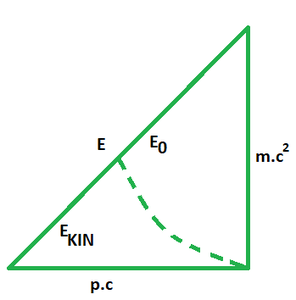Open In App

# Energy Momentum Formula

The energy-momentum relation is a relativistic equation that can be used to link an object’s mass, total energy, and momentum while it is at rest. This relativistic equation applies to a macroscopic body whose mass at rest is m0, the total energy is E, and momentum magnitude is p, with c denoting the speed of light as the constant. This equation applies to a system with total energy E, invariant mass m0, and momentum of size p; the constant c is the speed of light. It takes the special relativity scenario of flat spacetime into account. The total energy is the total of rest and kinetic energy, whereas invariant mass is mass measured in a center-of-mass frame.

In both of its meanings, the energy–momentum relationship is congruent with the well-known mass–energy relationship: E = mc2 describes the relationship between total energy E and (total) relativistic mass m (also known as mrel or mtot), whereas E0 = m0c2 describes the relationship between rest energy E0 and (invariant) rest mass m0.

### Energy Momentum Formula

The formula for the energy-momentum relation is given as follows,Where,

• E depicts the energy.
• p is the momentum of the object.
• c = speed of light
• m0 = rest mass

Derivation For energy-momentum formula

The energy-momentum relationship can be derived by blending the Einstein relationship with the relativistic momentum expression.E = mc2

p =⇒Hence proved.

### Sample Problems

Question 1: Find the momentum of a particle of mass 2 × 10-9 kg with 400 KJ energy.

Solution:

Given: E = 400 KJ and m0 = 2 × 10-9 kg

The formula for the energy- momentum relation isSubstituting the values in the formula we have,p = 119070.4 × 108 kg .m/s

Question 2: Find the velocity of a proton that has a momentum of 3 × 10−4 kg⋅m/s.

Solution:

Given: m = 1.67 × 10-27 kg and P = 3 × 10−4 kg⋅m/s

Since,Squaring both sides, we obtainu = 5.3 × 108 m/s

Question 3: At 12.0 V, a car battery is evaluated to shift 600 ampere-hours (Ah) of charge. Determine the increment in rest mass of the battery from fully drained to recharged, assuming that no chemical reactions take place.

Solution:

Given: Ebatt = (Δm)c2⇒Time needs to be converted into hours. Thus,Question 4: Find the velocity of a proton that has a momentum of 4.48 × 10−19 kg⋅m/s.

Solution:

Given: m = 1.67 × 10-27 kg and P = 4.48 × 10−19 kg⋅m/s

Since,Squaring both sides, we obtain⇒ u = 0.665996204 c

or, u = 1.997988 x 108 m/s

Question 5: Does an object with momentum always have energy? Is the reverse also true? Explain.

Solution:

Yes, an object with momentum always has energy. If the object has momentum (mv) it must be moving, and if it is moving it has kinetic energy.

No, an object with energy does NOT always have momentum. An object can be at rest and have potential energy (a book resting on a desk, for instance). Since this object’s velocity = 0, its momentum is zero.

Question 6: Find the velocity of an electron that has a momentum of 3.04 × 10−21 kg⋅m/s.

Solution:

Given: m = 9.11 × 10-31 kg and P = 4.48 × 10−19 kg⋅m/s

Since,Squaring both sides, we obtainQuestion 7: Find the velocity of an electron that has a momentum of 5 × 10−19 kg⋅m/s.

Solution:

Given: m = 9.11 × 10-31 kg and P = 5 × 10−19 kg⋅m/s

Since,Squaring both sides, we obtain: